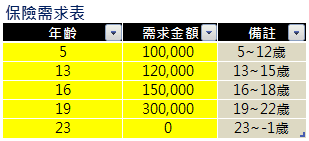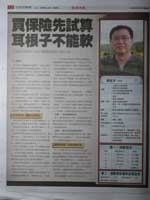﻿ 買保險先試算、耳根子不能軟

# 買保險先試算、耳根子不能軟## 步驟一：考慮通貨膨漲率

Excel公式：=目前物價*(1 + 通貨膨漲率)^年度

=100000*(1+2.2%)^0 = 100,000 => 5歲
=100000*(1+2.2%)^1 = 102,200 => 6歲
=100000*(1+2.2%)^2 = 104,448 => 7歲
……
=100000*(1+2.2%)^7 = 116,454 => 12歲

## 步驟二：算出未來金額的現值

Excel公式表示：=未來金額 / (1 + 投資報酬率)^年度

=116454/(1+5.0%)^7
=82,762

## 下載好用的試算表